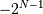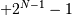# numpy.binary_repr¶

numpy.binary_repr(num, width=None)[source]

Return the binary representation of the input number as a string.

For negative numbers, if width is not given, a minus sign is added to the front. If width is given, the two’s complement of the number is returned, with respect to that width.

In a two’s-complement system negative numbers are represented by the two’s complement of the absolute value. This is the most common method of representing signed integers on computers [R16]. A N-bit two’s-complement system can represent every integer in the rangeto.

Parameters: num : int Only an integer decimal number can be used. width : int, optional The length of the returned string if num is positive, or the length of the two’s complement if num is negative, provided that width is at least a sufficient number of bits for num to be represented in the designated form. If the width value is insufficient, it will be ignored, and num will be returned in binary(num > 0) or two’s complement (num < 0) form with its width equal to the minimum number of bits needed to represent the number in the designated form. This behavior is deprecated and will later raise an error. Deprecated since version 1.12.0. bin : str Binary representation of num or two’s complement of num.

base_repr
Return a string representation of a number in the given base system.
bin
Python’s built-in binary representation generator of an integer.

Notes

binary_repr is equivalent to using base_repr with base 2, but about 25x faster.

References

 [R16] (1, 2) Wikipedia, “Two’s complement”, http://en.wikipedia.org/wiki/Two’s_complement

Examples

```>>> np.binary_repr(3)
'11'
>>> np.binary_repr(-3)
'-11'
>>> np.binary_repr(3, width=4)
'0011'
```

The two’s complement is returned when the input number is negative and width is specified:

```>>> np.binary_repr(-3, width=3)
'101'
>>> np.binary_repr(-3, width=5)
'11101'
```

#### Previous topic

numpy.set_string_function

numpy.base_repr